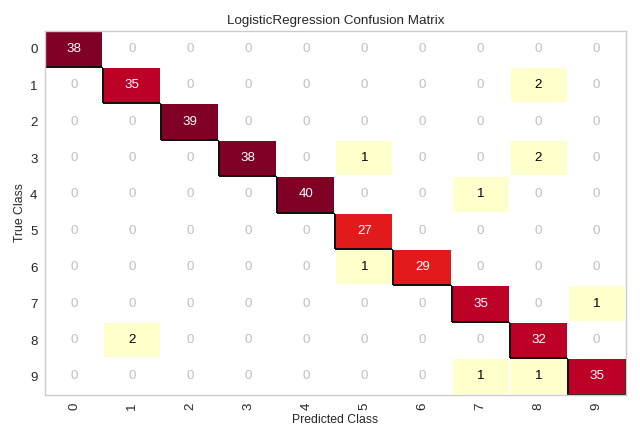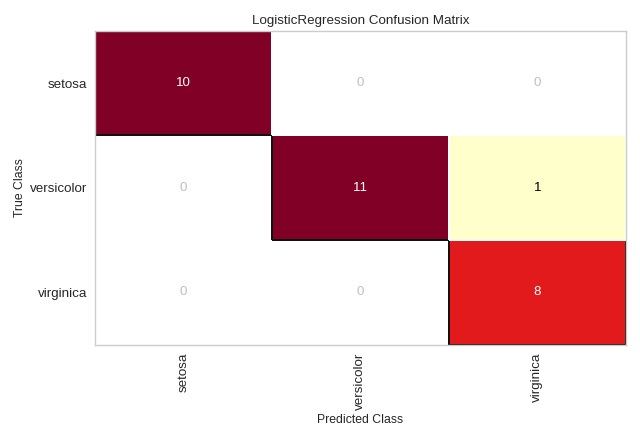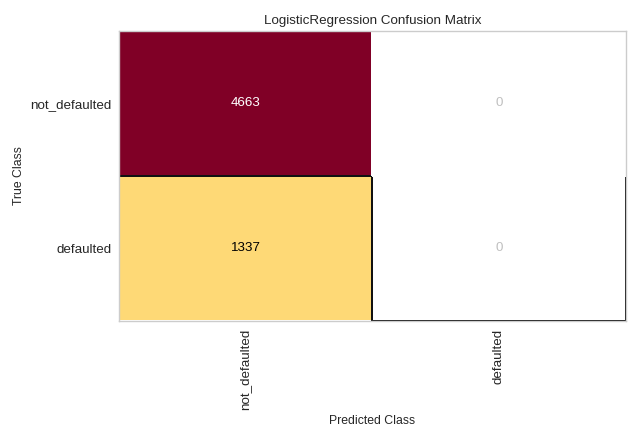# Confusion Matrix¶

The `ConfusionMatrix` visualizer is a `ScoreVisualizer` that takes a fitted scikit-learn classifier and a set of test `X` and `y` values and returns a report showing how each of the test values predicted classes compare to their actual classes. Data scientists use confusion matrices to understand which classes are most easily confused. These provide similar information as what is available in a `ClassificationReport`, but rather than top-level scores, they provide deeper insight into the classification of individual data points.

Below are a few examples of using the `ConfusionMatrix` visualizer; more information can be found by looking at the scikit-learn documentation on confusion matrices.

 Visualizer `ConfusionMatrix` Quick Method `confusion_matrix()` Models Classification Workflow Model evaluation
```from sklearn.datasets import load_digits
from sklearn.model_selection import train_test_split as tts
from sklearn.linear_model import LogisticRegression
from yellowbrick.classifier import ConfusionMatrix

# We'll use the handwritten digits data set from scikit-learn.
# Each feature of this dataset is an 8x8 pixel image of a handwritten number.
# Digits.data converts these 64 pixels into a single array of features
X = digits.data
y = digits.target

X_train, X_test, y_train, y_test = tts(X, y, test_size =0.2, random_state=11)

model = LogisticRegression(multi_class="auto", solver="liblinear")

# The ConfusionMatrix visualizer taxes a model
cm = ConfusionMatrix(model, classes=[0,1,2,3,4,5,6,7,8,9])

# Fit fits the passed model. This is unnecessary if you pass the visualizer a pre-fitted model
cm.fit(X_train, y_train)

# To create the ConfusionMatrix, we need some test data. Score runs predict() on the data
# and then creates the confusion_matrix from scikit-learn.
cm.score(X_test, y_test)

# How did we do?
cm.show()
```## Plotting with Class Names¶

Class names can be added to a `ConfusionMatrix` plot using the `label_encoder` argument. The `label_encoder` can be a sklearn.preprocessing.LabelEncoder (or anything with an `inverse_transform` method that performs the mapping), or a `dict` with the encoding-to-string mapping as in the example below:

```from sklearn.datasets import load_iris
from sklearn.linear_model import LogisticRegression
from sklearn.model_selection import train_test_split as tts

from yellowbrick.classifier import ConfusionMatrix

X = iris.data
y = iris.target
classes = iris.target_names

X_train, X_test, y_train, y_test = tts(X, y, test_size=0.2)

model = LogisticRegression(multi_class="auto", solver="liblinear")

iris_cm = ConfusionMatrix(
model, classes=classes,
label_encoder={0: 'setosa', 1: 'versicolor', 2: 'virginica'}
)

iris_cm.fit(X_train, y_train)
iris_cm.score(X_test, y_test)
iris_cm.show()
```## Quick Method¶

The same functionality above can be achieved with the associated quick method `confusion_matrix`. This method will build the `ConfusionMatrix` object with the associated arguments, fit it, then (optionally) immediately show it. In the below example we can see how a `LogisticRegression` struggles to effectively model the credit dataset (hint: check out `Rank2D` to examine for multicollinearity!).

```from yellowbrick.datasets import load_credit
from yellowbrick.classifier import confusion_matrix
from sklearn.linear_model import LogisticRegression
from sklearn.model_selection import train_test_split as tts

#Create the train and test data
X_train, X_test, y_train, y_test = tts(X, y, test_size=0.2)

# Instantiate the visualizer with the classification model
confusion_matrix(
LogisticRegression(),
X_train, y_train, X_test, y_test,
classes=['not_defaulted', 'defaulted']
)
plt.tight_layout()
```## API Reference¶

Visual confusion matrix for classifier scoring.

class yellowbrick.classifier.confusion_matrix.ConfusionMatrix(estimator, ax=None, sample_weight=None, percent=False, classes=None, encoder=None, cmap='YlOrRd', fontsize=None, is_fitted='auto', force_model=False, **kwargs)[source]

Bases: `yellowbrick.classifier.base.ClassificationScoreVisualizer`

Creates a heatmap visualization of the sklearn.metrics.confusion_matrix(). A confusion matrix shows each combination of the true and predicted classes for a test data set.

The default color map uses a yellow/orange/red color scale. The user can choose between displaying values as the percent of true (cell value divided by sum of row) or as direct counts. If percent of true mode is selected, 100% accurate predictions are highlighted in green.

Requires a classification model.

Parameters
estimatorestimator

A scikit-learn estimator that should be a classifier. If the model is not a classifier, an exception is raised. If the internal model is not fitted, it is fit when the visualizer is fitted, unless otherwise specified by `is_fitted`.

axmatplotlib Axes, default: None

The axes to plot the figure on. If not specified the current axes will be used (or generated if required).

sample_weight: array-like of shape = [n_samples], optional

Passed to `confusion_matrix` to weight the samples.

percent: bool, default: False

Determines whether or not the confusion_matrix is displayed as counts or as a percent of true predictions. Note, if specifying a subset of classes, percent should be set to False or inaccurate figures will be displayed.

classeslist of str, defult: None

The class labels to use for the legend ordered by the index of the sorted classes discovered in the `fit()` method. Specifying classes in this manner is used to change the class names to a more specific format or to label encoded integer classes. Some visualizers may also use this field to filter the visualization for specific classes. For more advanced usage specify an encoder rather than class labels.

encoderdict or LabelEncoder, default: None

A mapping of classes to human readable labels. Often there is a mismatch between desired class labels and those contained in the target variable passed to `fit()` or `score()`. The encoder disambiguates this mismatch ensuring that classes are labeled correctly in the visualization.

cmapstring, default: `'YlOrRd'`

Specify a colormap to define the heatmap of the predicted class against the actual class in the confusion matrix.

fontsizeint, default: None

Specify the fontsize of the text in the grid and labels to make the matrix a bit easier to read. Uses rcParams font size by default.

is_fittedbool or str, default=”auto”

Specify if the wrapped estimator is already fitted. If False, the estimator will be fit when the visualizer is fit, otherwise, the estimator will not be modified. If “auto” (default), a helper method will check if the estimator is fitted before fitting it again.

force_modelbool, default: False

Do not check to ensure that the underlying estimator is a classifier. This will prevent an exception when the visualizer is initialized but may result in unexpected or unintended behavior.

kwargsdict

Keyword arguments passed to the visualizer base classes.

Examples

```>>> from yellowbrick.classifier import ConfusionMatrix
>>> from sklearn.linear_model import LogisticRegression
>>> viz = ConfusionMatrix(LogisticRegression())
>>> viz.fit(X_train, y_train)
>>> viz.score(X_test, y_test)
>>> viz.show()
```
Attributes
classes_ndarray of shape (n_classes,)

The class labels observed while fitting.

class_counts_ndarray of shape (n_classes,)

Number of samples encountered for each class supporting the confusion matrix.

score_float

An evaluation metric of the classifier on test data produced when `score()` is called. This metric is between 0 and 1 – higher scores are generally better. For classifiers, this score is usually accuracy, but ensure you check the underlying model for more details about the metric.

confusion_matrix_array, shape = [n_classes, n_classes]

The numeric scores of the confusion matrix.

draw()[source]

Renders the classification report; must be called after score.

finalize(**kwargs)[source]

Finalize executes any subclass-specific axes finalization steps.

Parameters
kwargs: dict

generic keyword arguments.

Notes

The user calls show and show calls finalize. Developers should implement visualizer-specific finalization methods like setting titles or axes labels, etc.

score(X, y)[source]

Draws a confusion matrix based on the test data supplied by comparing predictions on instances X with the true values specified by the target vector y.

Parameters
Xndarray or DataFrame of shape n x m

A matrix of n instances with m features

yndarray or Series of length n

An array or series of target or class values

Returns
score_float

Global accuracy score

show(outpath=None, **kwargs)[source]

Makes the magic happen and a visualizer appear! You can pass in a path to save the figure to disk with various backends, or you can call it with no arguments to show the figure either in a notebook or in a GUI window that pops up on screen.

Parameters
outpath: string, default: None

path or None. Save figure to disk or if None show in window

clear_figure: boolean, default: False

When True, this flag clears the figure after saving to file or showing on screen. This is useful when making consecutive plots.

kwargs: dict

generic keyword arguments.

Notes

Developers of visualizers don’t usually override show, as it is primarily called by the user to render the visualization.

yellowbrick.classifier.confusion_matrix.confusion_matrix(estimator, X_train, y_train, X_test=None, y_test=None, ax=None, sample_weight=None, percent=False, classes=None, encoder=None, cmap='YlOrRd', fontsize=None, is_fitted='auto', force_model=False, show=True, **kwargs)[source]

Confusion Matrix

Creates a heatmap visualization of the sklearn.metrics.confusion_matrix(). A confusion matrix shows each combination of the true and predicted classes for a test data set.

The default color map uses a yellow/orange/red color scale. The user can choose between displaying values as the percent of true (cell value divided by sum of row) or as direct counts. If percent of true mode is selected, 100% accurate predictions are highlighted in green.

Requires a classification model.

Parameters
estimatorestimator

A scikit-learn estimator that should be a classifier. If the model is not a classifier, an exception is raised. If the internal model is not fitted, it is fit when the visualizer is fitted, unless otherwise specified by `is_fitted`.

X_trainarray-like, 2D

The table of instance data or independent variables that describe the outcome of the dependent variable, y. Used to fit the visualizer and also to score the visualizer if test splits are not specified.

y_trainarray-like, 2D

The vector of target data or the dependent variable predicted by X. Used to fit the visualizer and also to score the visualizer if test splits are not specified.

X_test: array-like, 2D, default: None

The table of instance data or independent variables that describe the outcome of the dependent variable, y. Used to score the visualizer if specified.

y_test: array-like, 1D, default: None

The vector of target data or the dependent variable predicted by X. Used to score the visualizer if specified.

axmatplotlib Axes, default: None

The axes to plot the figure on. If not specified the current axes will be used (or generated if required).

sample_weight: array-like of shape = [n_samples], optional

Passed to `confusion_matrix` to weight the samples.

percent: bool, default: False

Determines whether or not the confusion_matrix is displayed as counts or as a percent of true predictions. Note, if specifying a subset of classes, percent should be set to False or inaccurate figures will be displayed.

classeslist of str, defult: None

The class labels to use for the legend ordered by the index of the sorted classes discovered in the `fit()` method. Specifying classes in this manner is used to change the class names to a more specific format or to label encoded integer classes. Some visualizers may also use this field to filter the visualization for specific classes. For more advanced usage specify an encoder rather than class labels.

encoderdict or LabelEncoder, default: None

A mapping of classes to human readable labels. Often there is a mismatch between desired class labels and those contained in the target variable passed to `fit()` or `score()`. The encoder disambiguates this mismatch ensuring that classes are labeled correctly in the visualization.

cmapstring, default: `'YlOrRd'`

Specify a colormap to define the heatmap of the predicted class against the actual class in the confusion matrix.

fontsizeint, default: None

Specify the fontsize of the text in the grid and labels to make the matrix a bit easier to read. Uses rcParams font size by default.

is_fittedbool or str, default=”auto”

Specify if the wrapped estimator is already fitted. If False, the estimator will be fit when the visualizer is fit, otherwise, the estimator will not be modified. If “auto” (default), a helper method will check if the estimator is fitted before fitting it again.

force_modelbool, default: False

Do not check to ensure that the underlying estimator is a classifier. This will prevent an exception when the visualizer is initialized but may result in unexpected or unintended behavior.

show: bool, default: True

If True, calls `show()`, which in turn calls `plt.show()` however you cannot call `plt.savefig` from this signature, nor `clear_figure`. If False, simply calls `finalize()`

kwargsdict

Keyword arguments passed to the visualizer base classes.

Returns
vizConfusionMatrix

Returns the fitted, finalized visualizer